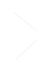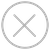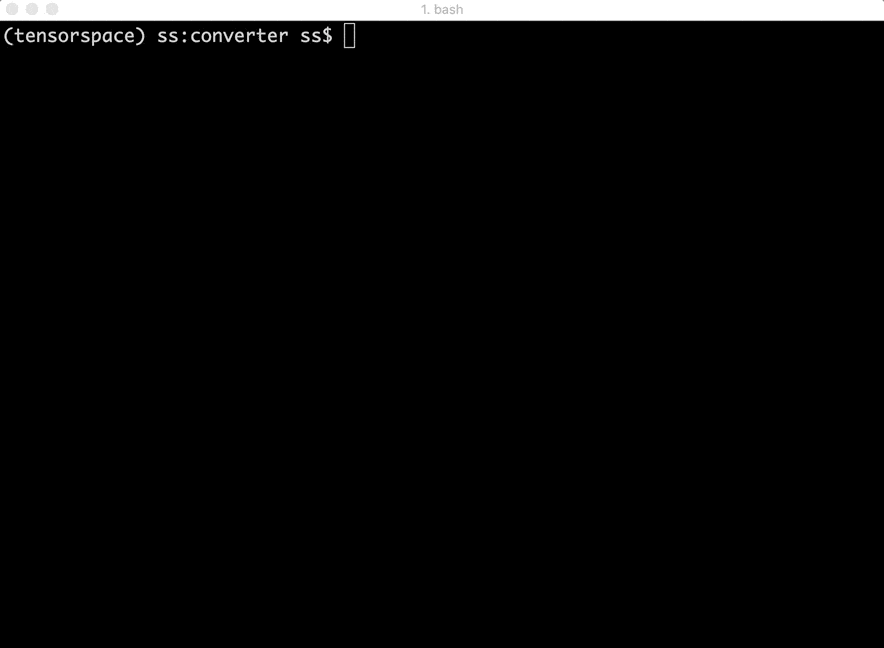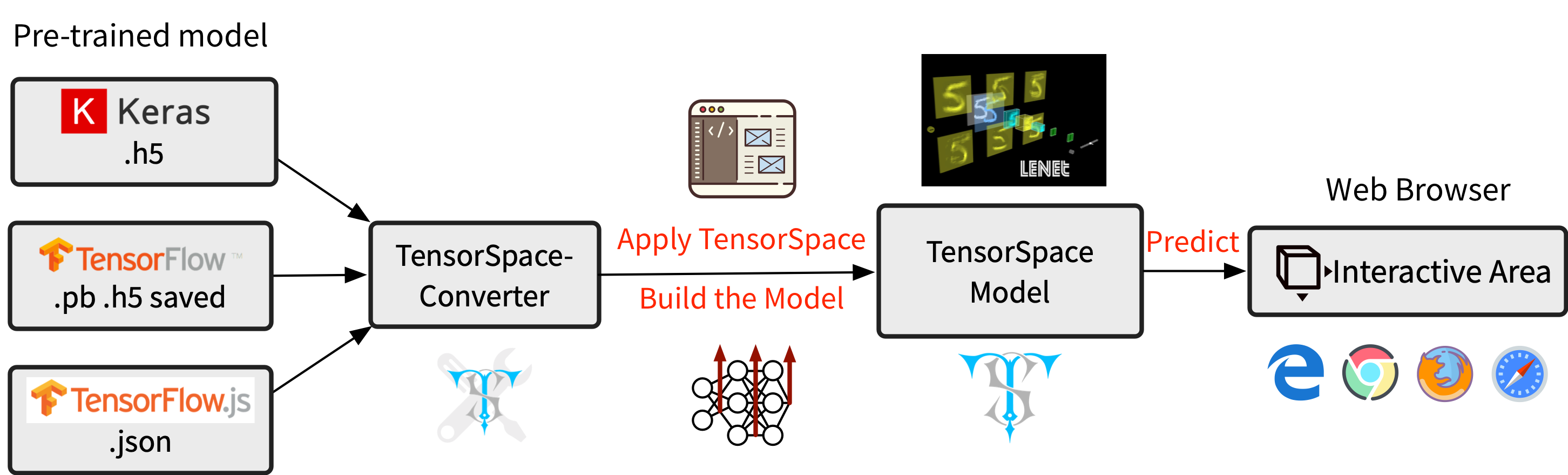TensorSpace.js
Getting StartBasic ConceptsTensorSpace ConverterModel PreprocessingModelsLayersMerge FunctionTensorSpace Converter
TensorSpace-Converter is a tool used to generate a TensorSpace compatible model from a pre-trained model built by TensorFlow, Keras and TensorFlow.js. TensorSpace-Converter includes the functions of: extracting information from hidden layers, matching intermediate data based on the configurations and exporting preprocessed TensorSpace compatible model.Fig. 1 - TensorSpace-Converter Usage
As a component of TensorSpace ecosystem, TensorSpace-Converter simplifies the TensorSpace preprocess, release the workloads from learning how to generate TensorSpace compatible model. As a development tool, TensorSpace-Converter helps to separate the work of model training and model visualization.Fig. 2 - TensorSpace-Converter Workflow